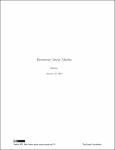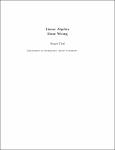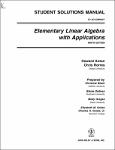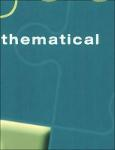### Search Results

Results 1-4 of 4 (Search time: 0.023 seconds).
• Previous
• 1
• Next
Item hits:BookElementary linear algebraAuthors: Kenneth Kuttler (2012)This is an introduction to linear algebra. The main part of the book features row operations and everything is done in terms of the row reduced echelon form and specific algorithms. At the end, the more abstract notions of vector spaces and linear transformations on vector spaces are presented. However, this is intended to be a first course in linear algebra for students who are sophomores or juniors who have had a course in one variable calculus and a reasonable background in college algebra. I have given complete proofs of all the fundamental ideas, but some topics such as Markov matrices are not complete in this book but receive a plausible introduction. The book contains a complete treatment of determinants and a simple proof of the Cayley Hamilton theorem although these are op...BookLinear Algebra Done WrongAuthors: Sergei Treil (2014)The title of the book sounds a bit mysterious. Why should anyone read this book if it presents the subject in a wrong way? What is particularly done “wrong” in the book? Before answering these questions, let me first describe the target audience of this text. This book appeared as lecture notes for the course “Honors Linear Algebra”. It supposed to be a first linear algebra course for mathematically advanced students. It is intended for a student who, while not yet very familiar with abstract reasoning, is willing to study more rigorous mathematics than what is presented in a “cookbook style” calculus type course. Besides being a first course in linear algebra it is also supposed to be a first course introducing a student to rigorous proof, formal definitions in short, to the style...BookElementary Linear Algebra with ApplicationsAuthors: Howard Anton (2005)This classic treatment of linear algebra presents the fundamentals in the clearest possible way, examining basic ideas by means of computational examples and geometrical interpretation. It proceeds from familiar concepts to the unfamiliar, from the concrete to the abstract. Readers consistently praise this outstanding text for its expository style and clarity of presentation. The applications version features a wide variety of interesting, contemporary applications. Clear, accessible, step-by-step explanations make the material crystal clear. Established the intricate thread of relationships between systems of equations, matrices, determinants, vectors, linear transformations and eigenvalues.BookLinear AlgebraAuthors: Harvey E. Rose (2002)In algebra, an entity is called linear if it can be expressed in terms of addition, and multiplication by a scalar; a linear expression is a sum of scalar multiples of the entities under consideration. Also, an operation is called linear if it preserves addition, and multiplication by a scalar. For example, if A and Bare 2 x 2 real matrices, v is a (row) vector in the real plane, and c is a real number, then v(A + B) = vA + vB and (cv)A = c(vA), that is, the process of applying a matrix to a vector is linear. Linear Algebra is the study of properties and systems which preserve these two operations, and the following pages present the basic theory and results of this important branch of pure mathematics. There are many books on linear algebra in the bookshops and libraries of the wor...